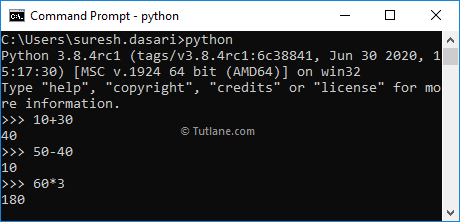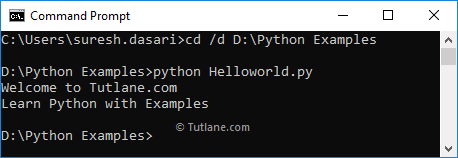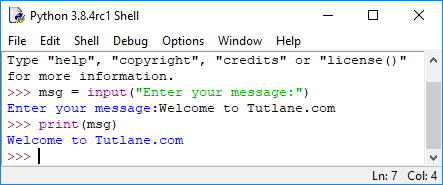# Python Basic Syntaxes

Here, you will learn how the basic syntaxes useful while writing the programs in a python programming language.

## Python Syntax Execution

As discussed in the Python Interpreter and Python Hello World chapters, you can execute the Python programs directly in the command-line interface or write the code in a script (`.py`) file and execute that file from the command line.

Following is the example of executing the python statements using the python command-line interface.Following is the example of executing the python script files using the command-line interface.## Python Keywords

In python, Keywords are the predefined set of reserved words that have special meaning for the compiler. So the keywords should not use as identifiers such as variable name, class name, etc., in our applications.

Following is the list of Python keywords that are available in the Python 3.8 version.

 False class from or None continue global pass True def if raise and del import return as elif in try assert else is while async except lambda with await finally nonlocal yield break for not

If you observe the above keywords, except `False`, `True` and `None` all other keywords are in lowercase, and we need to write them as they are because the keywords are case sensitive in python.

## Python Identifiers

In python, the Identifier is a name given to the programming elements like variables, classes, functions, etc., to differentiate one element from another.

While defining the identifiers in a python programming language, you need to follow specific naming convention rules.

• You can define the identifier with a combination of alphabets (lowercase, uppercase), numbers, and underscore (_). For example, the names. `abc`, `a2b`, `_abc` are valid ways to define the identifier.
• The identifier must always start with either alphabet or underscore but not with the numbers. For example, the name a2b is valid, but 2ab is invalid.
• Don't use any keywords as an identifier. For example, `global = 20` is invalid.
• The white space or special characters such as @, !, #, %, etc., are not allowed for an identifier. For example, `a b c = 20`, `a@b = 10` are invalid identifiers.
• In python, the identifier names are case sensitive, which means the name and Name are different.

## Python Variables

In python, variables are the storage locations to store the data in memory. In python, you can create the variables without specifying the type, and you need to follow the identifier naming convention rules.

Following is the example of creating the variables in python.

a = 100
b = "Welcome to Tutlane.com"
c = "Learn Python"

If you observe the above example, we created three variables (a, b, c) without specifying the variable type. As python is a type-inferred language, it will automatically decide the type based on the assigned value.

## Python Quotes

In python, quotes are useful to represent the string literals. You can use single (`'`), double (`"`), or triple (`'''`) quotes to denote the strings as long as the quotes' starting and ending are the same.

Following is the example of using different quotes in python to represent string literals.

a = 'Hi'
b = "Welcome to Tutlane"
c = '''Learn Python
with examples'''

If you observe the above example, we used different quotes (single, double, and triple) to represent the string literals.

The triple quotes (`'''`) are useful when you want to spread the single statement content to multiple lines.

## Python Statements

The Python Interpreter will treat each line of text as a statement. Following is the example of python statements.

a = 100
b = "Welcome to Tutlane.com"
c = "Learn Python"

In other programming languages, the semicolon (`;`) denotes the end of a statement, but in Python, the new line character will indicate the statement's end.

In case if you want to write a single statement by spreading the text to multiple lines, then you need to use the backslash (`\`) as a continuation character like as shown below.

a = 5 + 6 + 7 + \
7 + 7 + 1 + \
10 + 2 + 4
print(a)
b = "Welcome " \
"to Tutlane "\
"Learn Python with Examples"
print(b)

In python, you can also use parentheses `( )` to distribute the single statement text to multiple lines.

a = (5 + 6 + 7 +
7 + 7 + 1 +
10 + 2 + 4)
print(a)
b = ("Welcome "
"to Tutlane "
"Learn Python with Examples")
print(b)

In python, if you want to write the multiple statements in a single line, then use the semicolon (;) like as shown below.

a = 10; b = "Welcome to Tutlane"; c = "Learn Python"

## Python Indentation

In python, indentation indicates the block of code for control flow statements, class, and function definitions. The other programming languages, such as C#, JAVA, C/C++, will use braces `{ }` to define a block of code.

Generally, indentation means spaces at the beginning of the code. In other programming languages, indentation is just for code readability, and it’s optional, but in Python, indentation is significant to indicate the block of statements.

The following is a valid example of defining the If…Else statement with an indentation in python.

a = 10
b = 20
if a > b:
print("a is greater than b")
else:
print("b is greater than a") #output: b is greater than a

In python, if you skip the indentation, you will get an error. Following is the example of defining the If…Else statement in python without indentation. The following example will throw an exception because of missing the indentation in If…Else statement.

a = 10
b = 20
if a > b:
print("a is greater than b")
else:
print("b is greater than a") #IndentationError: expected an indented block

In Python, specifying the number of indentation spaces is up to you, but it has to be at least one. It must be consistent throughout the block. Otherwise, you will get IndentationError.

Following is the example of defining the If…Else statement in python with different indentations.

a = 10
b = 20
if a > b:
print("a is greater than b")
else:
print("b is greater than a")
print("b equals to a") #IndentationError: Unexpected indent

If you observe the above example, the else block does not have a consistent indentation. So, when we execute the above example, we will get an indentation error.

In python, comments are useful to provide information about the code that we wrote in our applications.

In Python, the hash (`#`) symbol is useful to indicate the comment line's start, as shown below.

#Python Variables
a = 10
b = 20
print("a is greater than b") #Print a value
print("a is greater than b") # Print b value

## Python Get User Input

In python, the `input()` function is useful to read the user input. The `input()` method will read the keystrokes and return them as a string object.

Following is the example of getting the user input using the python `input()` method.If you observe the above example, the `input()` method reads the user input ("`Welcome to Tutlane.com`") and assigns it to a `msg` variable.